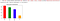# The art of Exploratory data analysis (EDA)

## What is Exploratory Data Analysis (EDA)?

As the name suggest, it is a technique to analyse the data by exploring it. EDA is one of the key as well as indispensable step in data analysis. This can be understood as a general checkup of patient (data) by the doctors (data enthusiast), before doing any surgery (analysis, modeling, prediction, classification, etc.).

## What are the goals of EDA?

EDA is done for two major underlying reasons, firstly to understand the data and secondly to identify faults or peculiar events (data points) in the dataset. Let’s try to understand what is meant by understanding and peculiar data points in detail.

• What is the high level contained information? (e.g cost data, revenue, budget, employee salaries, customer data, usage data and so on.)
• What are the variables available?
• What does their value mean? (e.g average time per page would mean that out of all the time spent by user on the website in one session, how much time was spent on a every particular page)
• What population segment data belong to? (e.g The data of cancer patient would meant that out of all patients the collected data is of cancer patients only.
• What is the Sample distribution?
• What is the strength and direction of the relationships among input variable and outcome variable?
• What is the Central tendency of the data?
• What is the spread of the data?
• Missing values
• Outliers (probable outliers or possible outliers)
• Assumption violations

# Part I — Categorical variables EDA

## How EDA is carried out?

As we have seen what and why of EDA, it time to answer how EDA is done? In this post we are going to focus on EDA techniques for categorical variables which includes ordinal, dichotomous and nominal variables. You can find details of types of variable here.

# Univariate categorical EDA

## Univariate-Quantitative EDA:

The most useful information that can be extracted from this analysis in context to the categorical variable, is to know the categories, frequency of occurrence, proportion or percentage of data falls under each category. Frequency tables are the most popular way of doing this analysis.

## Univariate-Graphical EDA:

When we think graphs, bar plots are one of the most widely used graphs. A similar information from frequency tabulation can also be attained in form of bar plots, which are more useful in presenting the analysis. Having able to present the information in visual form is one of the key element in effectively presenting the analysis.Bar plot to show total number of reservation on particular weekday

# Multivariate Categorical EDA

## Multivariate-Quantitative EDA:

1. Cross tabulation: Cross-tabulation is the basic bivariate non-graphical EDA technique. However, it is not limited to the bivariate, but can be extended further. Reason why more than 5-way cross tables are not very popular is because it becomes a little hard to apprehend as the cross tabulation grows.